Custom SearchDECIMALS When a decimal is raised to a power, the number of decimal places in the result is equal to the number of places in the decimal multiplied by the exponent. For example, consider (0.12). There are two decimal places in 0.12 and 3 is the exponent. Therefore, the number of places in the power will be 3(2) = 6. The result is as follows: (0.12)3 = 0.001728 The truth of this rule is evident when we recall the rule for multiplying decimals. Part of the rule states: Mark off as many decimal places in the product as there are decimal places in the factors together. If we carry out the multiplication, (0.12) x (0.12) x (0.12), it is obvious that there are six decimal places in the three factors together. The rule can be shown for any decimal raised to any power by simply carrying out the multiplication indicated by the exponent. Consider these examples: (1.4)2 = 1.96 (0.12)2 = 0.0144 (0.4)3 = 0.064 (0.02)2 = 0.0004 (0.2)2 = 0.04 Finding a root of a number is the inverse of raising a number to a power. To determine the number of decimal places in the root of a perfect power, we divide the number of decimal places in the radicand by the index of the root. Notice that this is just the opposite of what was done in raising a number to a power. Consider. The square root of 625 is 25. There are four decimal places in the radicand, 0.0625, and the index of the root is 2. Therefore, 4 + 2 = 2 is the number of decimal places in the root. We have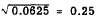Similarly,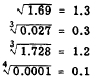LAWS OF EXPONENTS All of the laws of exponents may be developed directly from the definition of exponents. Separate laws are stated for the following five cases: 1. Multiplication. 2. Division. 3. Power of a power. 4. Power of a product. 5. Power of quotient. MULTIPLICATION To illustrate the law of multiplication, we examine the following problem: 43 x 42 = ? Recalling that 43 means 4 x 4 x 4 and 42 means 4 x 4, we see that 4 is used as a factor five times. Therefore 43 x 42 is the same as 45. This result could be written as follows: 43 x 42 = 4 x 4 x 4 x 4 x 4 = 45 Notice that three of the five 4’s came from the expression 43, and the other two 4’s came from the expression 42. Thus we may rewrite the problem as follows: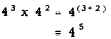The law of exponents for multiplication may be stated as follows: To multiply two or more powers having the same base, add the exponents and raise the common base to the sum of the exponents. This law is further illustrated by the following examples:Common Errors It is important to realize that the base must be the same for each factor, in order to apply the laws of exponents. For example, 23 x 32 is neither 25 nor 35. There is no way to apply the law of exponents to a problem of this kind. Another common mistake is to multiply the bases together. For example, this kind of error in the foregoing problem would imply that 23 x 32 is equivalent to 65, or 7776. The error of this may-be proved as follows:DIVISION The law of exponents for division may be developed from the .following example: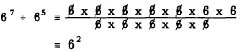Cancellation of the five 6’s in the divisor with five of the 6’s in the dividend leaves only two 6’s, the product of which is 62. This result can be reached directly by noting that 62 is equivalent to 6(7 - 5). In other words, we have the following: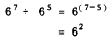Therefore the law of exponents for division is as follows: To divide one power into another having the same base, subtract the exponent of the divisor from the exponent of the dividend. Use the number resulting from this subtraction as the exponent of the base in the quotient. Use of this rule sometimes produces a negative exponent or an exponent whose value is 0. These two special types of exponents are discussed later in this chapter. POWER OF A POWER Consider the example (32)4. Remembering that an exponent shows the number of times the base is to be taken as a factor and noting in this case that 32 is considered the base, we have (32)4 = 32 x 32 x 32 x 32 Also in multiplication we add exponents. Thus, 32 x 32 x 32 x 32= 3(2+2+2 +2) = 38 Therefore,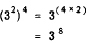The laws of exponents for the power of a power may be stated as follows: To find the power of a power, multiply the exponents. It should be noted that this case is the only one in which multiplication of exponents is performed. POWER OF A PRODUCT Consider the example (3 x 2 x 5)3. We know that (3 x 2 x 5)3 = (3 x 2 x 5)(3 x 2 x 5)(3 x 2 x 5) Thus 3, 2, and 5 appear three times each as factors, and we can show this with exponents as 33, 23, and 53. Therefore,  (3 x 2 x 5)3 = 32 x 23 x 53 The law of exponents for the power of a product is as follows: The power of a product is equal to the product obtained when each of the original factors is raised to the indicated power and the resulting powers are multiplied together.